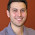## Monday, November 9, 2009

### DBSCAN clustering algorithm

DBSCAN is a well-known clustering algorithm, which is easy to implement. Quoting Wikipedia: "Basically, a point q is directly density-reachable from a point p if it is not farther away than a given distance ε (i.e., is part of its ε-neighborhood), and if p is surrounded by sufficiently many points such that one may consider p and q be part of a cluster.... For practical considerations, the time complexity is mostly governed by the number of getNeighbors queries. DBSCAN executes exactly one such query for each point, and if an indexing structure is used that executes such a neighborhood query in O(logn), an overall runtime complexity of$O(n \cdot \log n)$ is obtained."

1. DBScan does not require you to know the number of clusters in the data a priori, as opposed to k-means.
2. DBScan can find arbitrarily shaped clusters.
3. DBScan has a notion of noise.
4. DBScan requires just two parameters and is mostly insensitive to the ordering of the points in the database
1. DBScan needs to materialize the distance matrix for finding the neighbords. It has a complexity of O((n2-n)/2) since only an upper matrix is needed. Within the distance matrix the nearest neighbors can be detected by selecting a tuple with minimums functions over the rows and columns. Databases solve the neighborhood problem with indexes specifically designed for this type of application. For large scale applications, you cannot afford to materialize the distance matrix
2. Finding neighbords is an operation based on distance (generally the Euclidean distance) and the algorithm may find the curse of dimensionality problem

Here you have a DBSCAN code implemented in C++, boost and stl

1.Thanks, for sharing the code.
That would be nice to see how the DBSCAN will work if one would use the local Adaptive Metric(Mahalanobis metric) for the distance instead of Euclidean distance.
I am trying to implement it for 6D(x,y,z,vx,vy,vz)

thanks
Arman.

2.Thanks for releasing the code. However; could you specify the license under which it is released?

Thanks

3.Hi,
Thank you for sharing the codes. However, I met a problem when I try to compile the codes with visual studio 2005. I had installed boost on my computer, but it still does not work out. Can you please show me some possible solutions to this problem?

Thank you so much!

4.hi,

Thank you for sharing the codes. However, I met a problem when I tried to compile the codes in visual studio. Then, I installed boost on my computer. However, it does not work out. Could you please show me some possible solutions to this problem?

Thank you so much!

5.At lines 16 and 33 in distance.h I had to change

typedef typename VEC_T vector_type;
to
typedef VEC_T vector_type;

to get it to compile.

Dave

6.The example is clustering random numbers into 1 cluster. Actually would be good if the example was in some way illustrative of this method - on a more "shapy" data - like in the paper on DBSCAN.

7.actually I tried this code on ELKI example, it produced just one cluster, where ELKI gave several clusters with the same parameters.

8.thanks for sharing the code..where I can find real dataset for applying DBSCAN?

9.Hi

I've found a very very small bug in your code.
in clusters.cpp file

in findNeighbours function:
if ((pid != j ) && (_sim(pid, j)) > threshold)

it's supposed to be less than
if ((pid != j ) && (_sim(pid, j)) < threshold)

after changing this it works like charm, thanks for the code

10.Hi,

Thanks for sharing this code. The last commenter (M^3 Team) 's correction is wrong. Similarity, not distance is being compared! I wrote this small extra bit of code to provide the example where one can see several clusters.

void randomInit_twopopulations (Points & ps, unsigned int dims,
unsigned int num_points)
{
for (unsigned int j = 0; j < num_points; j++)
{
Point p(dims);

if (j < num_points/2)